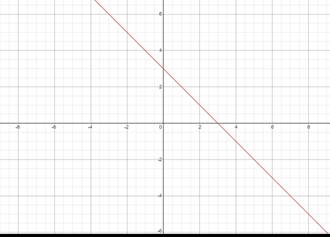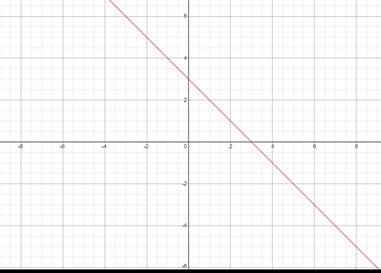# Slope and y-intercept of given equation, plot its graph### Precalculus: Mathematics for Calcu...

6th Edition
Stewart + 5 others
Publisher: Cengage Learning
ISBN: 9780840068071### Precalculus: Mathematics for Calcu...

6th Edition
Stewart + 5 others
Publisher: Cengage Learning
ISBN: 9780840068071

#### Solutions

Chapter 1.10, Problem 45E
To determine

## Slope and y-intercept of given equation, plot its graph

Expert Solution

Slope: -1

y-intercept: 3### Explanation of Solution

Given information:

x+y=3

Formula used:

Slope-intercept form of line: y=mx+c , where m is the slope and c is the y-intercept cut by the line.

x+y=3

Converting the line into slope intercept form

y=x+3So,m=1c=3

Plotting the Graph

Finding two points on line

x=11+y=3y=2(1,2)

x=00+y=3y=3(0,3)### Have a homework question?

Subscribe to bartleby learn! Ask subject matter experts 30 homework questions each month. Plus, you’ll have access to millions of step-by-step textbook answers!